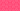PricingResources

# What is terminal value?

Written by

Also referred to as the horizon value or continuing value, terminal value is an important financial metric that you’ll need to know if you’re forecasting future cash flows. Find out a little more about how to do a terminal value calculation with our definitive guide.

## Terminal value explained

So, what is terminal value? Essentially, terminal value refers to the present value of all your business’s cash flows at a future point, assuming a stable rate of growth in perpetuity. It’s used for a broad range of financial metrics, but most prominently, terminal value is used to calculate discounted cash flow (DCF). So, for anyone who needs to do a DCF calculation, terminal value is vital.

## Why is terminal value significant?

It becomes more challenging to forecast value the further into the future you attempt to look. This is especially true for something as volatile as a cash flow. However, it’s imperative to value businesses and assets as effectively as possible, which is why financial models like discounted cash flow are used to calculate the total value of a project/business. Terminal value is one of the two primary components of discounted cash flow, and as such, it’s likely to play a crucial role in any forecasting attempts made by your firm.

## Terminal value calculation methods

There are two methods used for terminal value calculations:

• Terminal multiple method – Also referred to as the exit multiple method, the terminal multiple method assumes a finite window of operations. As such, the terminal value will need to reflect the net realisable value of the business’s assets at this time. In most cases, the terminal multiple method assumes that the business will be sold at the end of the projection period. To complete this terminal value calculation, you’ll multiply a statistic (the terminal multiple) like EBITDA by a projected statistic (i.e., the relevant statistic projected in the previous year). The terminal value formula for the terminal multiple method is as follows:

• Perpetuity growth model – Unlike the terminal multiple method, the perpetuity growth model assumes that your business’s cash flow values will grow at a steady rate ad infinitum. To do this, you’ll divide the last cash flow forecast by the difference between the terminal growth rate and the discount rate. The terminal value formula for the perpetuity growth model is as follows:

Where:

• Free Cash Flow = FCF from the last 12 months

• WACC = Weighted Average Cost of Capital

• g = Perpetuity growth rate

## Disadvantages of using a terminal value formula

There are a couple of limitations associated with both terminal value formulas mentioned above. For the terminal multiple method, it’s important to remember that terminal multiples are dynamic and change over time. When it comes to the perpetuity growth model, it’s tough to project an accurate rate of growth. At the same time, any assumed values used in the formula can lead to inaccuracies with your terminal value calculation.

Of course, these limitations don’t mean that terminal value isn’t a meaningful metric. However, they do indicate that it’s essential to use a wide range of multiples and applicable rates to ensure that you’re getting an acceptable result.

## We can help

GoCardless helps you automate payment collection, cutting down on the amount of admin your team needs to deal with when chasing invoices. Find out how GoCardless can help you with ad hoc payments or recurring payments.